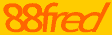# The Concept of Asset Depreciation - Part 2

Written by Farhaa Xha for Gaebler Ventures

Straight -- line depreciation is the most frequently used and the simplest method of calculating and recording depreciation.

This theory assumes that an asset provides equal benefits each year and should be depreciated in equal amounts until it reaches its salvage or scrap value. And the great news is we only need three numbers to ascertain the value of deprecation; the original cost of the asset, its estimated useful life and the salvage value.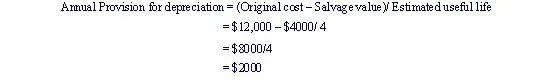For each of the four years these assets are active, a depreciation charge of \$2,000 will be made.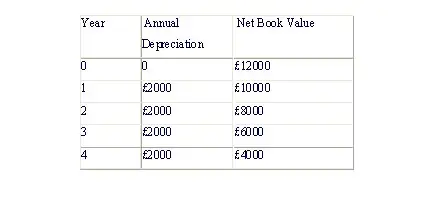Why it is called the straight line method? It's simple - if you plot the data on a graph (y axis represents value and x axis represents year or date) it appears like this, YES! A straight line.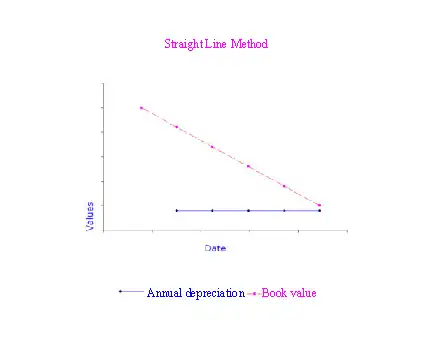Straight line method assumes that fixed asset provides the same service and/or is used in similar regularity.

However, some assets are used more in the initial years (e.g. trucks, computers etc) and used less in subsequent years as it gets inefficient and old. Similarly, when the economic activity is low we use it less frequently.

As with most methods, we use 'estimated figures' regarding both scrap value and life expectancy. Mistakes in these figures may lead to misleading charges made to depreciation.

Reducing Balance Method (RBM)

Reducing Balance Method is expected to be used on an asset when it generates more benefits in the early years of its life, than in its following years. Instead of charging constant rate of depreciation each year, RBM charges high expense in its early years and declines more dramatically in its subsequent years. RBM allocates a fixed percentage of the book value as an annual expense. We arrive at our book value by deducting each year's depreciation from the previous book value. The book value will equal the original estimated scrap value.

How do I calculate RBM?

There are few ways to calculate depreciation % rate. Calculating depreciation percentage can pose problems as it is hard to determine the appropriate percentage. Let's have look at a simple calculation.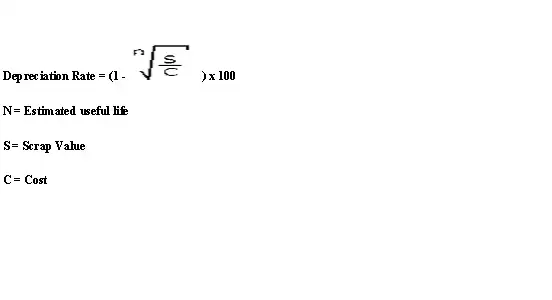Take an example:

An asset costing \$15,000 depreciated at 25% a year will be depreciated by \$3,750 in the first year, but by 25% of the residual (i.e. \$15,000 - \$3,750) in the second year. In comparison to straight-line method, depreciation is more greatly weighted towards early years.

How do these methods impact my profit?

Reducing Balance Method reduces profit in the early years of an asset's life but improve them in the following whereas straight line method has equal impact on the profit figure.

It is more realistic to use RBM for some kind of assets such as motor vehicle as it reflects that asset of this nature lose more value in their early years than in later ones.

Net Book Value is not essentially equal to the current market value of the asset as the NBV is based on forecasted figures.

At the end of it's useful year, if the asset is sold for more than their expected scrap value than we can deduce it was 'over depreciated.'

When assets are sold at a surplus, we record the difference on income statement as a 'profit on disposal of asset.'

When should I start and end depreciating my assets?

One cannot record 'depreciation' of an asset unless it is used for productive purpose i.e. purchased but not used as yet.

Charging depreciation expense should stop when the asset's useful life is over. It requires making a prediction on how long we expect an asset would add to revenue generation and based on that we estimate the 'useful life of an asset' and its value upon disposal. In addition, depreciation expense must be stopped when the book value equals the residual value, when it seizes to be a functional part of your business, when you sell off your assets. (There are also accounting rules on starting and ending depreciation to ensure standardization and accuracy.)

It is possible to depreciate different assets using different methods depending on its nature and the needs of your business.

Farhaa is a student pursuing a concentration in Economics and Finance at London school of Economics and Political Science, University of London. She is passionate about making accounting and economics accessible to entrepreneurs. Farhaa will also be sharing unconventional yet effective ways of recruiting.

## Conversation Board

We greatly appreciate any advice you can provide on this topic. Please contribute your insights on this topic so others can benefit.